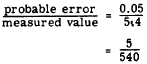Custom SearchPercent of Error The accuracy of a measurement is determined by the RELATIVE ERROR. The relative error is the ratio between the probable error and the quantity being measured. This ratio is simply the fraction formed by using the probable error as the numerator and the measurement itself as the denominator. For example, suppose that a metal plate is found to be 5.4 inches long, correct to the nearest tenth of an inch. The maximum probable error is five hundredths of an inch (one-half of one tenth of an inch) and the relative error is found as follows:Thus the relative error is 5 parts out of 540. Relative error is usually expressed as PERCENT OF ERROR, When the denominator of the fraction expressing the error ratio is divided into the numerator, a decimal is obtained. This decimal, converted to percent, gives the percent of error. For example, the error in the foregoing problem could be stated as 0.93 percent, since the ratio 5/540 reduces to 0.0093 (rounded off) in decimal form. Significant Digits The accuracy of a measurement is often described in terms of the number of significant digits used in expressing it. If the digits of a number resulting from measurement are examined one by one, beginning with the left-hand digit, the first digit that is not 0 is the first significant digit. For example, 2345 has four significant digits and 0.023 has only two significant digits. The digits 2 and 3 in a measurement such as 0.023 inch signify how many thousandths of an inch comprise the measurement. The Os are of no significance in specifying the number of thousandths in the measurement; their presence is required only as "place holders" in placing the decimal point. A rule that is often used states that the significant digits in a number begin with the first nonzero digit (counting from left to right) and end with the last digit. This implies that 0 can be a significant digit if it is not the first digit in the number. For example, 0.205 inch is a measurement having three significant digits. The 0 between the 2 and the 5 is significant because it is a part of the number specifying how many hundredths are in the measurement. The rule stated in the foregoing paragraph fails to classify final 0s on the right. For example, in a number such as 4,700, the number of significant digits might be two, three, or four. lf the 0s merely locate the decimal point (that is, if they show the number to be approximately forty-seven hundred rather than forty seven), then the number of significant digits is two. However, if the number 4,700 represents a number such as 4,730 rounded off to the nearest hundred, there are three significant digits. The last 0 merely locates the decimal point. If the number 4,700 represents a number such as 4,700.4 rounded off, then the number of significant digits is four. Unless we know how a particular number was measured, it is sometimes impossible to determine whether right-hand Os are the result of rounding off. However, in a practical situation it is normally possible to obtain information concerning the instruments used and the degree of precision of the original data before any rounding was done. In a number such as 49.30 inches, it is reasonable to assume that the 0 in the hundredths place would not have been recorded at all if it were not significant. In other words, the instrument used for the measurement can be read to the nearest hundredth of an inch. The 0 on the right is thus significant. This conclusion can be reached another way by observing that the 0 in 49.30 is not needed as a place holder in placing the decimal point. Therefore its presence must have some other significance. The facts concerning significant digits may be summarized as follows: 1. Digits other than 0 are always significant. 2. Zero is significant when it falls between significant digits. 3. Any final 0 to the right of the decimal point is significant. 4. When a 0 is present only as a place holder for locating the decimal point, it is not significant. 5. The following categories comprise the significant digits of any measurement number: a. The first nonzero left-hand digit is significant. b. The digit which indicates the precision of the number is significant. This is the digit farthest to the right, except when the right-hand digit is 0. If it is 0, it may be only a place holder when the number is an integer. c. All digits between significant digits are significant. Practice problems. Determine the percent of error and the number of significant digits in each of the following measurements: 1. 5.4 feet 2. 0.00042 inch 3. 4.17 set 4. 147.50 miles Answers: 1. Percent of error: 0.93%     Significant digits: 2 2. Percent of error: 1.19%     Significant digits: 2 3. Percent of error: 0.12%     Significant digits: 3 4. Percent of error: 0.0034%     Significant digits: 5Integrated Publishing, Inc. - A (SDVOSB) Service Disabled Veteran Owned Small Business